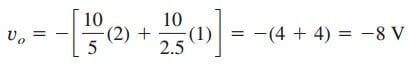# Simple Summing Amplifier Equation and ExplanationNow we will move on to the next topic: “Summing Amplifier Equation’ along with its easy equation.

## Summing Amplifier Equation

Besides amplification, the op amp can perform addition and subtraction. The addition is performed by the summing amplifier covered in this section; the subtraction is performed by the difference amplifier covered in the next section.

A summing amplifier is an op amp circuit that combines several inputs and produces an output that is the weighted sum of the inputs.

The summing amplifier, shown in Figure.(1), is a variation of the inverting amplifier. It takes advantage of the fact that the inverting configuration can handle many inputs at the same time.

We keep in mind that the current entering each op amp input is zero. Applying KCL at node a gives

But

We note that va = 0 and substitute Equation.(2) into Equation.(1). We get

indicating that the output voltage is a weighted sum of the inputs. For this reason, the circuit in Figure.(1) is called summer. Needless to say, the summer can have more than three inputs.

## Summing Amplifier Equation Example

Calculate vo and io in the op amp circuit in Figure.(2).The current io is the sum of the currents through the 10- and 2-kΩ resistors. Both of these resistors have voltage vo = −8 V across them, since va = vb = 0. Hence,